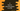# C program to save the output of a program to file## How to save the output of a C program to a file:

In this post, we will learn how to save the output of a C program to a file. Note that this is only for GCC/G++. So, if you are working on any unix machine, you can follow these steps.

### Let’s write a program:

Let’s write a program that will print all even values from 1 to 100.

``````#include <stdio.h>

int main()
{
int i;

for (i = 1; i <= 100; i++)
{
if (i % 2 == 0)
{
printf("%d ", i);
}
}

return 0;
}``````

This is a simple program that uses a for loop to print the even numbers.

• This loop runs from 1 to 100
• On each iteration, it checks if it can divide the current value by 2 or not. It uses modulo operator % to check the remainder if we divide the number by 2. If it is 0, it is a even number.
• The printf is printing the even numbers.

### Compile and run the program:

If you are using gcc, you can compile it by using the below command:

``gcc example.c``

We are assuming that the program is saved in a file example.c.

It will create one new file a.out. Now, we need to run this file to get the output. Use the below command for that:

``./a.out``

It will execute the program and it will print the output on the terminal.

There is a way to print the output to a file. This works with any other terminal command as well. You just need to do:

``command > filename``

In our case the command is ./a.out and we can add any filename.

For example,

``./a.out > output.txt``

It will create a new file output.txt in the same folder and write the output of the program to this file.

Note that it will not print the content on terminal if you are writing them to a file.